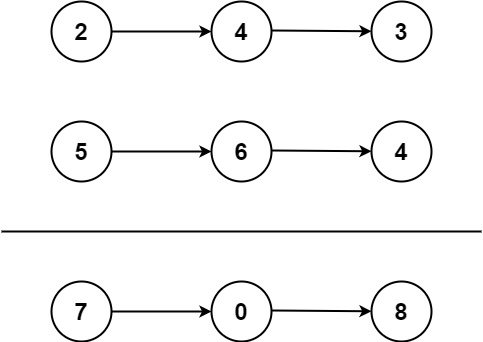# Add Two Numbers LeetCode problem SolutionAdd Two Numbers LeetCode problem Solution

In this post, we are going to Add Two Numbers LeetCode problem which is related to arrays and Hash Table. Let’s have a look at the problem statement first and then try to solve the problem.

## Add Two Numbers LeetCode Problem Statement

You are given two non-empty linked lists representing two non-negative integers. The digits are stored in reverse order, and each of their nodes contains a single digit. Add the two numbers and return the sum as a linked list.

You may assume the two numbers do not contain any leading zero, except the number 0 itself.

Example 1:```Input: l1 = [2,4,3], l2 = [5,6,4]
Output: [7,0,8]
Explanation: 342 + 465 = 807.
```

Example 2:

```Input: l1 = , l2 = 
Output: 
```

Example 3:

```Input: l1 = [9,9,9,9,9,9,9], l2 = [9,9,9,9]
Output: [8,9,9,9,0,0,0,1]
```

Constraints:

• The number of nodes in each linked list is in the range `[1, 100]`.
• `0 <= Node.val <= 9`
• It is guaranteed that the list represents a number that does not have leading zeros.

## Add Two Numbers LeetCode Problem Solutions

I will Provide solution in Multiple programming languages for you. If you are not able to find the code in required language then please share in comments so that our team can help you.

### Problem Solution in C++

``````class Solution {
public:
ListNode* addTwoNumbers(ListNode* l1, ListNode* l2) {
ListNode* dummy=new ListNode();
ListNode* temp=dummy;
int carry=0;
while(l1!=NULL || l2!=NULL || carry){
int sum=0;
if(l1!=NULL){
sum+=l1->val;
l1=l1->next;
}
if(l2!=NULL){
sum+=l2->val;
l2=l2->next;
}
sum+=carry;
carry=sum/10;
ListNode* newnode=new ListNode(sum%10);
temp->next=newnode;
temp=temp->next;
}
return dummy->next;
}
};``````

### Problem Solution in Python

``````class Solution:
def addTwoNumbers(self, l1: ListNode | None, l2: ListNode | None) -> ListNode | None:
p, q, curr_sum = l1, l2, sum_head
carry = 0
while p or q or carry:
p, p_val = (p.next, p.val) if p else (p, 0)
q, q_val = (q.next, q.val) if q else (q, 0)
carry, sum_ = divmod(p_val + q_val + carry, 10)
curr_sum.next = ListNode(sum_)
curr_sum = curr_sum.next

### Problem Solution in JavaScript

``````var addTwoNumbers = function(l1, l2) {
let values = [];
let overflow = 0;
while (l1.next || l2.next) {
const value = (l1.val + l2.val + overflow) % 10;
overflow = l1.val + l2.val + overflow >= 10 ? 1 : 0;
values.push(value);

l1 = l1.next || new ListNode(0);
l2 = l2.next || new ListNode(0);
}
const value = (l1.val + l2.val + overflow) % 10;
overflow = l1.val + l2.val + overflow >= 10 ? 1 : 0;
values.push(value);
if (overflow === 1) {
values.push(1);
}
let node = undefined;
values.reverse().forEach(item => {
if (node === undefined) {
node = new ListNode(item);
} else {
const newNode = new ListNode(item);
newNode.next = node;
node = newNode;
}
});
return node;
};``````